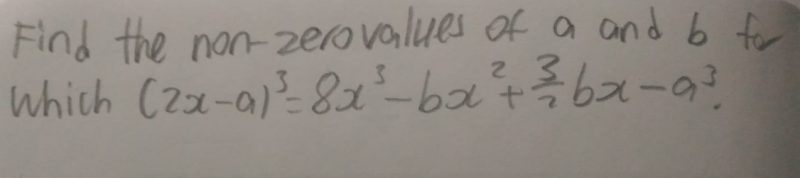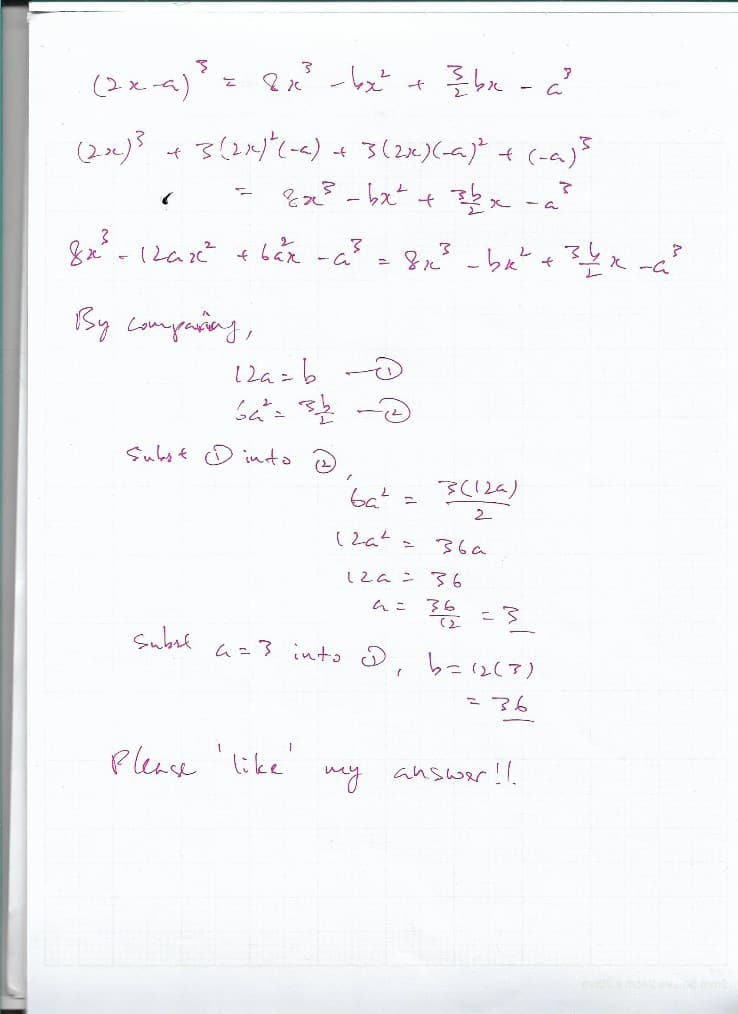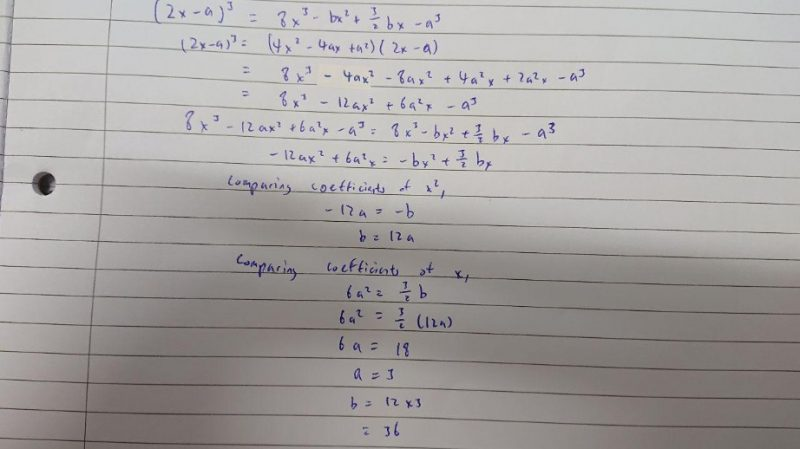# Questionsec 3 Amath question on binomial theorem

3 Answers

# Answer0 Replies 1 Like ✔Accepted Answer

(2x – a)^3 = (2x)^3 + 3[(2x)^2](-a) + 3(2x)(-a)^2 + (-a)^3= 8x^3 – 12a(x^2) + 6(a^2)x – a^3 = 8x^3 – bx^2 + (3/2)bx – a^3
By comparing,
12a = b ——- (1)
6a^2 = (3/2)b ——- (2)
Substituting (1) into (2), we get
6a^2 = (3/2)(12a) = 18a
a = 3
Substituting a = 3 into (1),
b = 36

Ans : a = 3 and b = 36.

0 Replies 0 LikesWe hope this was helpful for you! We are a group of university students trying to build an education startup and we would be very grateful if you could visit our website and leave any comments/feedback you have. Thank you!

http://www.learnwithsherpa.com/

0 Replies 0 Likes